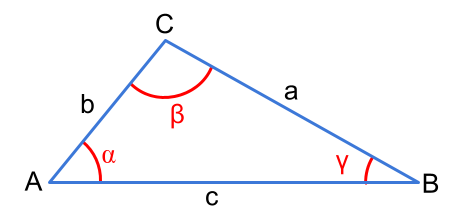Question #692

# What is the definition of the of the cosine formula?Statement of the law of cosines (also known as the al-Kashi's theorem): the square of any one side of a triangle is equal to the difference between the sum of squares of the other two sides and double the product of other sides and cosine angle included between them.

In the above triangle, we can apply the law of cosines on each side :

﻿﻿

﻿﻿

﻿﻿

18 events in history

Icons proudly provided by Friconix.